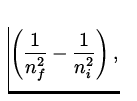Atomic Spectra

When one heats up a gas, it emits light of various wavelengths. For monatomic gases formed of only one kind of atom the emission spectra contains only light of particular wavelengths, and for hydrogen the spectra obeys a very simple relation:= RH(1)

where ni and nf are positive non-zero integers with ni > nf and RH is a constant called Rydberg's constant:

 RH = 1.097 x 107 m - 1. (2)

One of the first such series of lines discovered which obey Eq.(28.1) was found by Balmer, which corresponded to nf = 2 and is in the visible light region.

Many models of the hydrogen atom were proposed to explain this and other simple properties. One of the more notable ones was a planetary model'' due to Rutherford, where a single electron orbits around the proton, much like the earth orbits around the sun. However, there were many shortcomings of this model, the main one being the fact that the electron, being charged, is predicted to emit radiation as it accelerates around the proton. The electron turns out to lose energy in this way at a very rapid rate, and the model predicts a lifetime of a hydrogen atom of around 10- 10 s, which is obviously incorrect.Next: The Bohr Model Up: Atomic Physics Previous: Atomic Physics